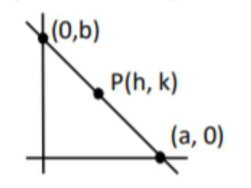# A man is walking on a straight line.

Question:

A man is walking on a straight line. The arithmetic mean of the reciprocals of the intercepts of this line on the coordinate axes is $\frac{1}{4}$. Three stones $A, B$ and $C$ are placed at the points $(1,1),(2,2)$ and $(4,4)$ respectively. Then which of these stones is/are on the path of the man?

1. (1) B only

2. (2) A only

3. (3) All the three

4. (4) $\mathrm{C}$ only

Correct Option: 1

Solution:

$\frac{x}{a}+\frac{y}{b}=1$

$\frac{h}{a}+\frac{k}{b}=1 \ldots$ (1)

$\frac{\frac{1}{a}+\frac{1}{b}}{2}=\frac{1}{4} \ldots(2)$

$\therefore \frac{1}{a}+\frac{1}{b}=\frac{1}{2}$

$\therefore$ Line passes through fixed point $\mathrm{B}(2,2)$

(from (1) and (2))